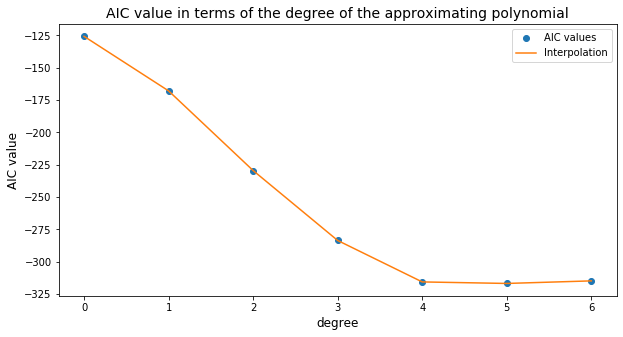# Choosing the Best Fit with AIC¶

In this final section, we use the Akaike Information Criterion (AIC) (see Burnham \& Anderson (2002); Bender (2000)) to select the most efficient polynomial approximation.

## Importing the libraries¶

In :
import csv
import numpy as np
import matplotlib.pyplot as plt


## Akaike Information Criterion¶

Beink $k$ the number of parameters in the model, $N$ the number of data points in the sample, and $E$ the mean quadratic error with the approximation with $k$ parameters, the Akaike Information Criterion (AIC) is given by

$$\text{AIC} = N\ln(E) + 2k.$$

The first term decreases with $E$, but the second term penalizes a high number of parameters.

There are a number of other criteria, such as Bayesian information criterion (BIC), Mallow's $C_p$, AICc (for a low number of samples), adjusted $R^2$; ridge regression; and cross validation.

### AIC for the approximating polynomials¶

In our case, a polynomial model of degree $j$ has $k=j+1$ parameters. Denoting $E_j$ as the mean quadratic error associated with this polynomial, we find the $\text{AIC}_j$ for each polynomial as

$$\text{AIC}_j = N\ln(E_j) + 2(j+1).$$

For the computation of the $\text{AIC}_j$ values, we first load the data from file.

This time, we use csv to read only the header of the file, contained in the first two rows, and then we use numpy to read the temperature and density values, which are in the remaining rows. This is a more efficient way to load the data.

We start by reading the header. We read the first two lines of the file and make a dictionary from it.

In :
water_csv_reader = csv.reader(open('water.csv',"r"), delimiter=",")
header = dict([(line1[i], line2[i]) for i in range(3)])

Out:
{'temp': 'Temperature (C)',
'density': 'Density (g/cm^3)',
'viscosity': 'Viscosity (cm^2/s)'}

Next we read the data values for temperature and density, which are in the first and second columns, so we skip the first two rows and ignore the last column.

In :
T, f = np.loadtxt(open('water.csv', "r"), delimiter=",", skiprows=2, usecols=(0,1), unpack=True)


We also define two auxiliary variables related to the number of rows.

In :
N = len(T)
N_half = int(N/2)


### Computing the mean quadratic error¶

With the data in memory, we compute the $\text{AIC}_j$ values.

In :
A = list()
Err = list()
for j in range(N_half):
A.append(np.vstack([T**i for i in range(j+1)]).T)
Err.append(np.linalg.lstsq(A[j], f, rcond=None)/N)
print(f'j={j}: Error={Err[j]:.2e}')

j=0: Error=1.75e-04
j=1: Error=9.22e-06
j=2: Error=1.33e-07
j=3: Error=3.16e-09
j=4: Error=3.27e-10
j=5: Error=2.64e-10
j=6: Error=2.64e-10


### Computing the AIC values¶

In :
AIC = [len(T)*np.log(Err[j]) + 2*(j+2) for j in range(N_half)]
for j in range(len(AIC)):
print(f'j={j}: AIC={AIC[j]:.2f}')

j=0: AIC=-125.74
j=1: AIC=-167.91
j=2: AIC=-229.52
j=3: AIC=-283.59
j=4: AIC=-315.63
j=5: AIC=-316.81
j=6: AIC=-314.83


### Plotting the AIC value¶

Finally, we plot the AIC values in terms of the degree of the polynomials.

In :
plt.figure(figsize=(10,5))
plt.plot(range(len(AIC)),AIC, 'o', color='tab:blue', label='AIC values')
plt.plot(range(len(AIC)), AIC, color='tab:orange', label='Interpolation')
plt.legend()
plt.xlabel('degree', fontsize=12)
plt.ylabel('AIC value', fontsize=12)
plt.title('AIC value in terms of the degree of the approximating polynomial', fontsize=14)
plt.show()